# Concept of Polyhedron

## Definition

• Polyhedron: A polyhedron is a closed space figure whose faces are polygons. A polyhedron is said to be regular if its faces are made up of regular polygons and the same number of faces meet at each vertex.
• Platonic solids: A regular polyhedron is made up of regular polygons. Such solids are also known as ‘platonic solids’.
• Non-polyhedron: A non-polyhedron is not a closed space figure, maybe one or more of the sides is a curved surface.

## Notes

### Polyhedron:

• A polyhedron is a closed space figure whose faces are polygons.

• The word polyhedron has Greek origins, meaning many faces.

• A solid figure consisting of four or more plane faces (all polygons), pairs of which meet along an edge, three or more edges meeting at a vertex.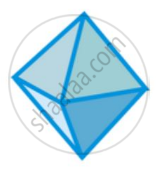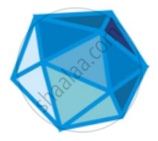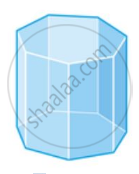• Each of these solids is made up of polygonal regions which are called its faces; these faces meet at edges which are line segments, and the edges meet at vertices which are points. Such solids are called polyhedrons.

#### Types of the polyhedron:

• A polyhedron is said to be regular if its faces are made up of regular polygons and the same number of faces meet at each vertex. any polyhedron that does not meet these conditions is considered irregular.

### Irregular Polyhedron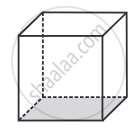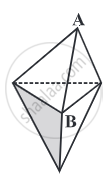This polyhedron is regular. Its faces are congruent, regular polygons. Vertices are formed by the same number of faces.

This polyhedron is not regular. All the sides are congruent, but the vertices are not formed by the same number of faces. 3 faces meet at A but 4 faces meet at B.

## Regular polyhedra:

A regular polyhedron is a polyhedron whose faces are all congruent, regular polygons. A regular polyhedron is named based on its number of faces. There are only five polyhedra that are regular polyhedra; these are referred to as Platonic solids.

### Number of faces

Tetrahedron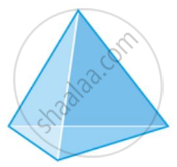4

Cube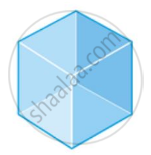6

Octahedron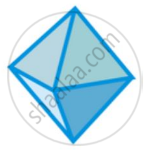8

Dodecahedron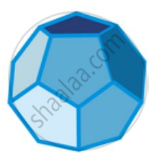12

Icosahedron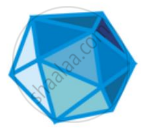20

In the above table, each regular polyhedra is named based on its number of faces. The net below each sketch shows a 2D picture of all of the faces of the polyhedron.

## Non-Polyhedrons:

A non-polyhedron is not a closed space figure, maybe one or more of the sides is a curved surface. Thus, Solids with curved faces are called Non-polyhedrons.
Example: Sphere, Cylinder, Cone, etc.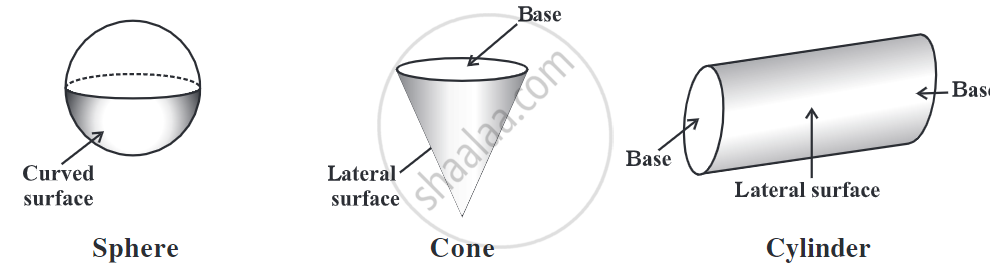## Concave and Convex polyhedrons:

• Convex Polyhedron: If the surface of a polyhedron does not intersect itself and the line segment connecting any two points of the polyhedron lies within its interior part or surface then such a polyhedron is a convex polyhedron.
• Concave Polyhedron: A non-convex polyhedron is termed as a concave polyhedron.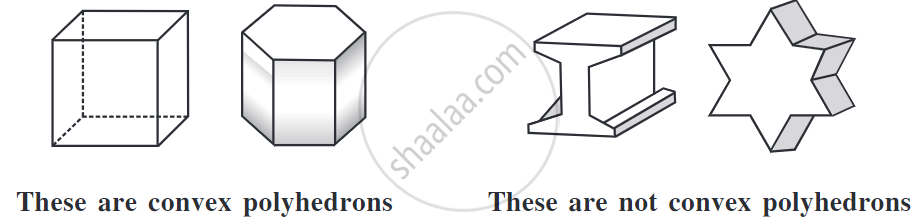If you would like to contribute notes or other learning material, please submit them using the button below.

### Shaalaa.com

What are Polyhedrons? [00:12:59]
S
0%